Geometry

Area of a triangle

Area of a triangle - formulas and calculator to calculate the area of a triangle. Triangle area - is a numerical characteristic characterizing the size of the plane bounded by a geometric figure formed by three segments (sides) that connect three points (vertices) that are not lying on one straight line.

Different formulas are used to calculate the area of a triangle, depending on the known input data. Below are formulas and a calculator that can help you calculate the area of a triangle or check the calculations that have already been done. General formulas for all types of triangles are given, special cases for equilateral, isosceles and right triangles.

Our calculator for calculating the area will help you calculate the area of different types of triangles or check the already performed calculations.

For all triangles

1

Area of triangle by its base and height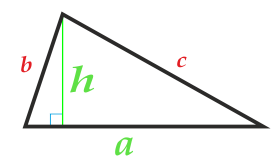Area of triangle is equal to half the product of the base of the triangle by the height dropped on this base: . The triangle's base can be chosen from either side of the triangle.

Side a
Height h

2

Area of triangle on two sides and the angle between them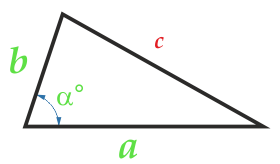Area of the triangle is equal to half the product of any two of its sides by the sine of the angle between these sides: . The angle α between the sides can be anything: blunt, sharp, straight.

Side a
Side b
Angle α° between parties a and b

3

Area of triangle along the radius of the inscribed circle and the three sides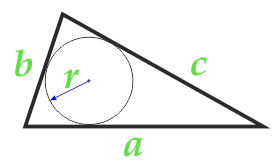Area of triangle is equal to half the sum of all three sides of the triangle multiplied by the radius of the inscribed circle.  or in another way you can say: Area of the triangle is equal to half the perimeter of the triangle multiplied by the radius of the inscribed circle.

Side a
Side b
Side c

4

Area of triangle along the radius of the circumscribed circle and the three sides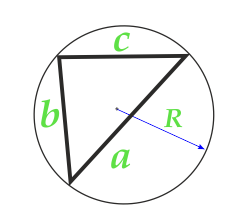Area of triangle is equal to the product of three sides of the triangle divided by four radii of the circumscribed circle:

Side a
Side b
Side c
Radius R of the circumscribed circle

5

Area of triangle according to Heron's formula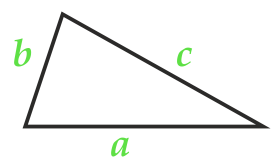If you know all three sides of a triangle, you can calculate its area using the Heron formula: , where p is the half -perimeter of the triangle, calculated by formula

Side a
Side b
Side c
Semi-perimeter:

For isosceles triangles

6

Area of an isosceles triangle along the sides and the angle between themCalculate area:

Side a (a = b)
Angle α° between the sides

7

Area of an isosceles triangle on the side, base and angle between them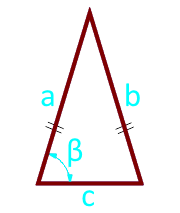Calculate area:

Side a (a = b)
The base of the triangle c
Angle β° between base and side

8

Area of an isosceles triangle on the base and angle between the sidesCalculate area:

The base of the triangle c
Angle α° between the sides

For equilateral triangles

9

Area of an equilateral triangle on the side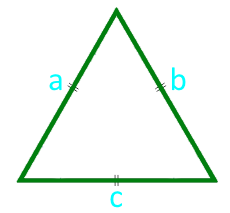Calculate area:

Side a (a = b = c)

10

Area of an equilateral triangle in height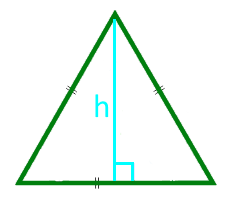Calculate area:

Height h

11

Area of an equilateral triangle along the radius of the inscribed circle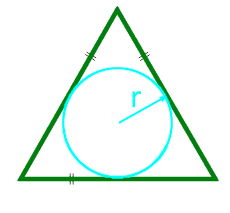Calculate area:

12

Area of an equilateral triangle along the radius of the circumscribed circle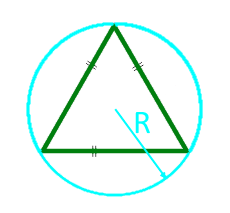Calculate area:

Radius R of the circumscribed circle

For right-angled triangles

13

Square of a right triangle with two legs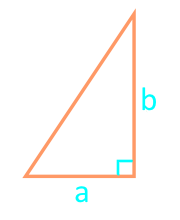Calculate area:

Cathete a
Cathete b

14

Area of a right-angled triangle along the segments dividing the hypotenuse into an inscribed circle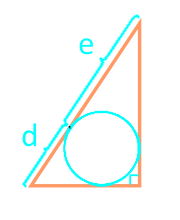Calculate area:

Line segment d
Line segment e

15

Area of a right-angled triangle according to Heron's formula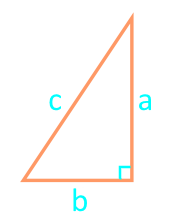Heron's formula for a right triangle , where p is the half -perimeter of the triangle, calculated by formula

Calculate area:

Side a
Side b
Side c

Semi-perimeter:

Definitions

A triangle is a geometric figure formed by three segments that connect three points that are not lying on one straight line. The segments are called the sides of the triangle, and the points are the vertices of the triangle.

Area - is a numerical characteristic characterizing the size of a plane bounded by a closed geometric figure.

Area is measured in units of measurement squared: km2, m2, cm2, mm2, etc.

Depending on the type of triangle and its known source data, the area of the triangle can be calculated:

For all triangles

For isosceles triangles

For equilateral triangles

For right-angled triangles